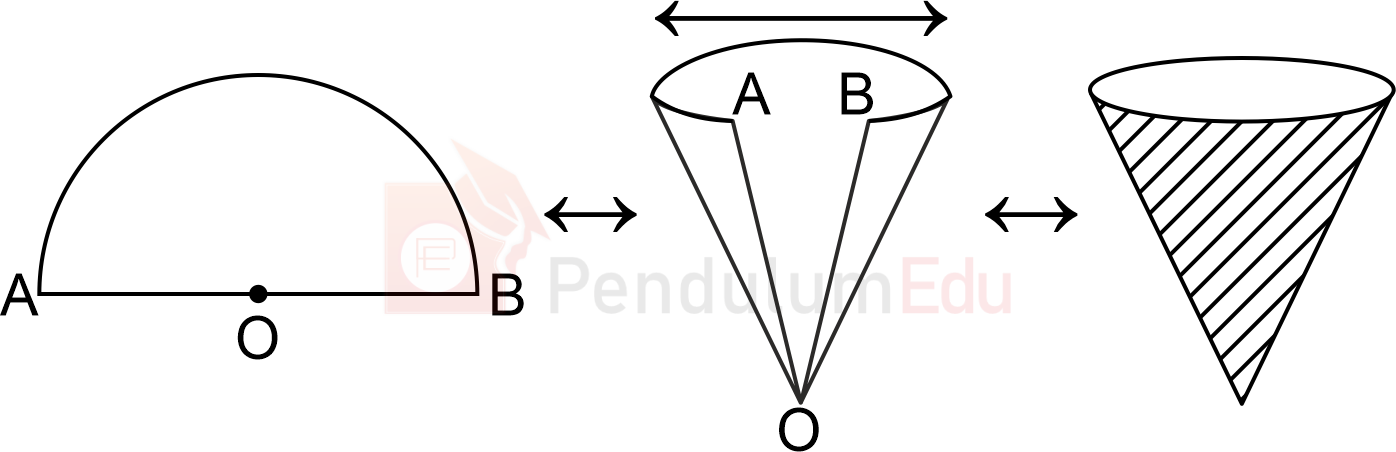Question of The Day21-06-2021

A semicircular sheet of radius 14 cm is rolled up into the cone in a way that two binding radii are joined together. Find the total surface area of the cone formed?

Correct Answer : a ) 308 cm2

Explanation :

According to the question

A semicircular sheet of radius 14 cm is rolled up into a cone

Let the radius of the cone be xWhen the semicircular sheet is rolled up to form the cone, then the Radius becomes the slant height of the cone and the circumference of the circle becomes the circumference of the base of the Cone.

⇒Slant height (l) = 14 cm

We know that

Circumference of the semicircle = πr

$${22 \over 7}*14$$=44 cm

⇒ Perimeter of the base of the cone = 2πr

$$2*{22 \over 7}*x=44$$

⇒ x = 7 cm

Since the cone will be a hollow cone from the bottom.

Total surface are of the cone=$$πxl$$

Where x is the radius of the cone and l is the slant height

$${22 \over 7}*7*14$$

⇒22*21

⇒308 cm2

Hence, (a) is the correct answer.0